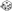Race Class Ability Scores Dice Roll: 1d6z d6 Results: 2(2) Dice Roll: 1d9z d9 Results: 9(9) 1 = Human 2 = Elf 3 = Dwarf 4 = Halfling 5 = Gnome 6 = Half-Elf 1 = Fighter 2 = Ranger 3 = Paladin 4 = Cleric 5 = Druid 6 = Thief 7 = Bard 8 = Mage 9 = Illusionist Dice Roll: 3d6z d6 Results: 5, 1, 1 (Total = 7)Strength (7) Dice Roll: 3d6z d6 Results: 2, 6, 5 (Total = 13)Dexterity (13) Dice Roll: 3d6z d6 Results: 3, 4, 4 (Total = 11)Constitution (11) Dice Roll: 3d6z d6 Results: 4, 3, 2 (Total = 9)Intelligence (9) Dice Roll: 3d6z d6 Results: 3, 2, 3 (Total = 8)Wisdom (8) Dice Roll: 3d6z d6 Results: 3, 1, 2 (Total = 6)Charisma (6)

A dumb yet dexterous elven illusionist. Perhaps a stage magician, who does sleight of hand illusions.

And again...

 Race Class Ability Scores Dice Roll: 1d6z d6 Results: 4(4) Dice Roll: 1d9z d9 Results: 8(8) 1 = Human 2 = Elf 3 = Dwarf 4 = Halfling 5 = Gnome 6 = Half-Elf 1 = Fighter 2 = Ranger 3 = Paladin 4 = Cleric 5 = Druid 6 = Thief 7 = Bard 8 = Mage 9 = Illusionist Dice Roll: 3d6z d6 Results: 3, 6, 5 (Total = 14)Strength (14) Dice Roll: 3d6z d6 Results: 4, 2, 4 (Total = 10)Dexterity (10) Dice Roll: 3d6z d6 Results: 4, 4, 1 (Total = 9)Constitution (9) Dice Roll: 3d6z d6 Results: 6, 4, 4 (Total = 14)Intelligence (14) Dice Roll: 3d6z d6 Results: 1, 5, 1 (Total = 7)Wisdom (7) Dice Roll: 3d6z d6 Results: 6, 3, 5 (Total = 14)Charisma (14)

A strong, intelligent, charismatic halfling mage who is quite impulsive.